# Find the inverse, if it exists, of the given matrix 1 0 0 OA. 0 1...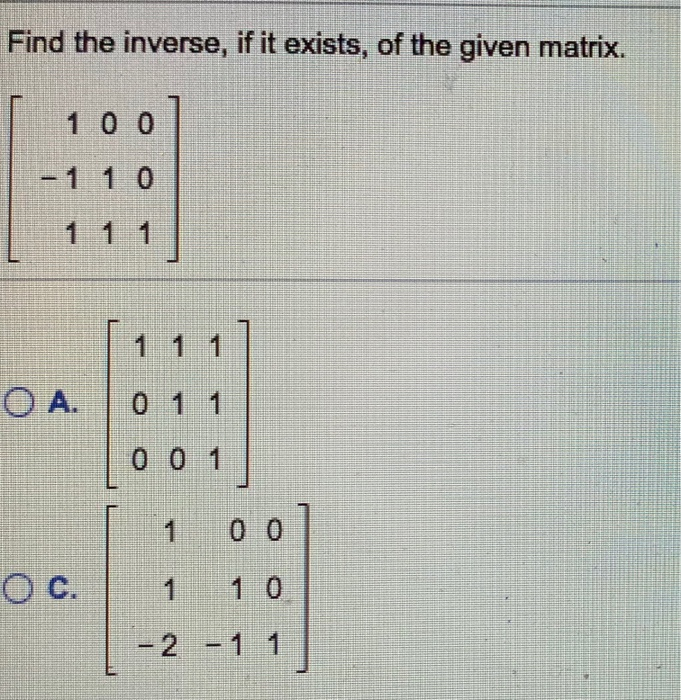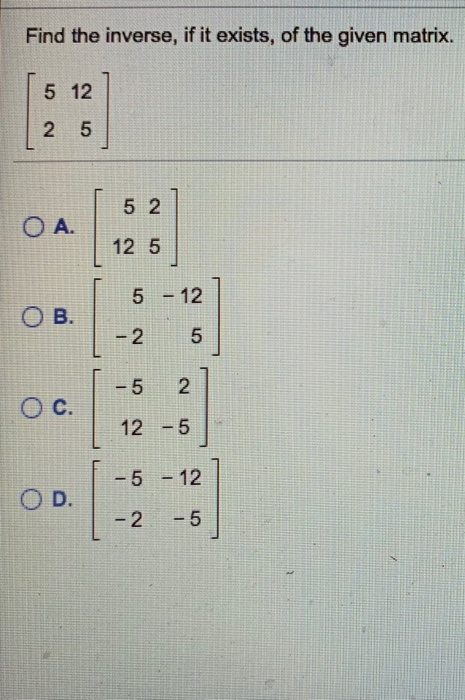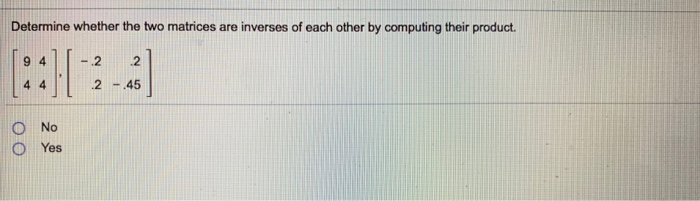Find the inverse, if it exists, of the given matrix 1 0 0 OA. 0 1 1 0 0 1 1 0 0 2-1 1
Find the inverse, if it exists, of the given matrix. 5 12 5 2 A. 12 5 5 -12 -2 5 -5 2 12 -5 -5-12 -25 OB. O c. O D.
Determine whether the two matrices are inverses of each other by computing their product. 9 4-22 2 -45 O No O Yes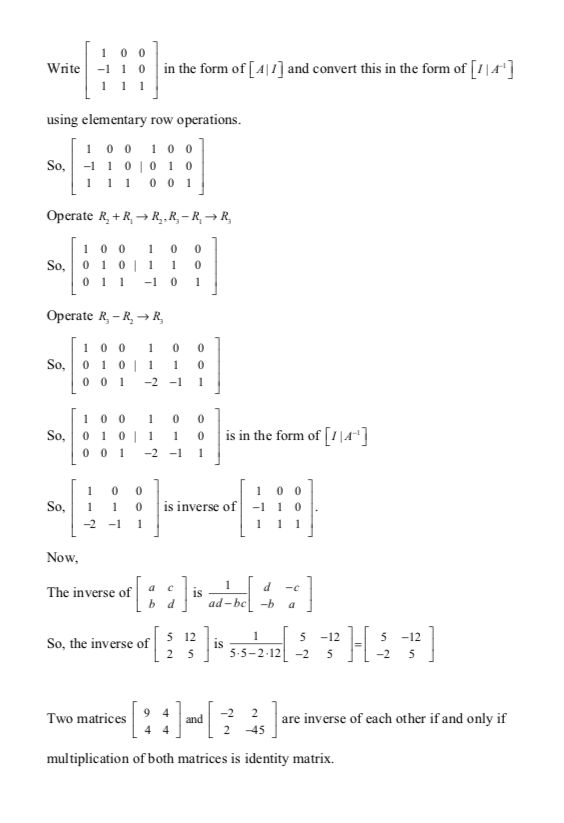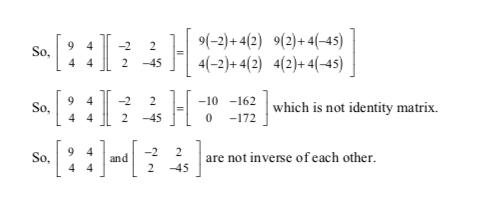#### Earn Coin

Coins can be redeemed for fabulous gifts.

Similar Homework Help Questions
• ### Use a graphing utility to find the inverse, if it exists, of the given matrix. Select...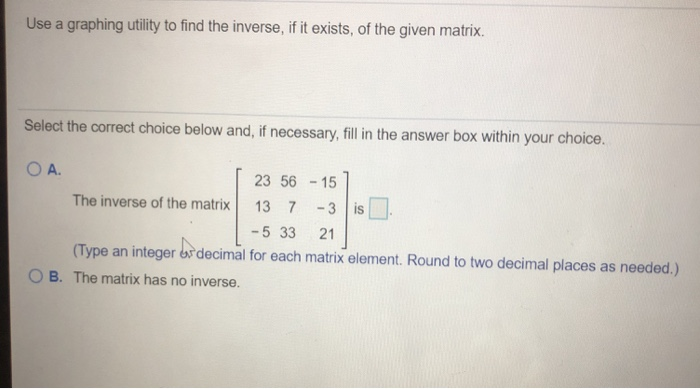Use a graphing utility to find the inverse, if it exists, of the given matrix. Select the correct choice below and, if necessary, fill in the answer box within your choice. OA. 23 56 - 15 The inverse of the matrix 13 7 -3 is . - 5 33 21 (Type an integer os decimal for each matrix element. Round to two decimal places as needed.) OB. The matrix has no inverse.

• ### 3. (10 points) Simultaneous left inverse The two matrices 3 2] and both left-invertible, and have multiple left inverses. Do they have a common left inverse? Explain how to find a 2 × 4 matrix C that...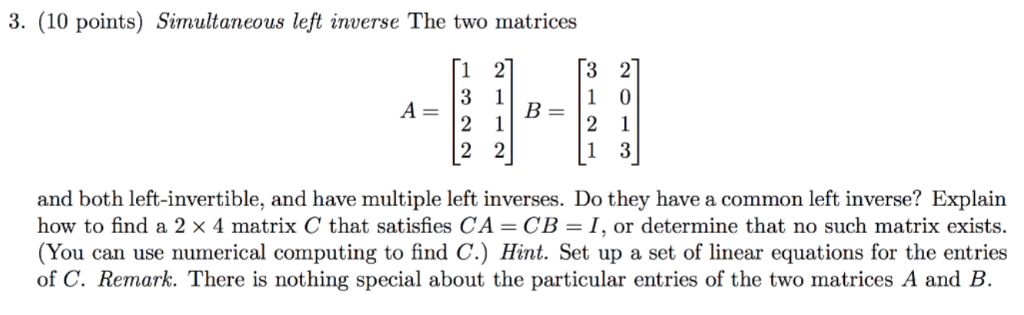3. (10 points) Simultaneous left inverse The two matrices 3 2] and both left-invertible, and have multiple left inverses. Do they have a common left inverse? Explain how to find a 2 × 4 matrix C that satisfies CA-CB-1, or determine that no such matrix exists. (You can use numerical computing to find C.) Hint. Set up a set of linear equations for the entries of C. Remark. There is nothing special about the particular entries of the two matrices...

• ### Decide whether or not the matrices are inverses of éach other. and 0 1 -110 10...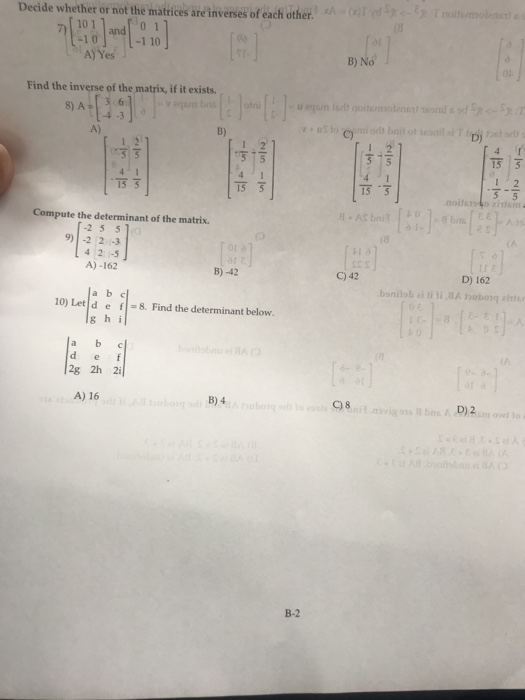Decide whether or not the matrices are inverses of éach other. and 0 1 -110 10 A]Yes' 」 B) No Find the inverse of the matrix, if it exists. 8) A36 A) B) C) D) T5亏 15 5 15可 15 3 Compute the determinant of the matrix. 2 5 5 9) -2 2 -3 4 2 -5 A)-162 B)-42 C) 42 D) 162 a b c 10) Let d ef g h i 8. Find the determinant below. a b...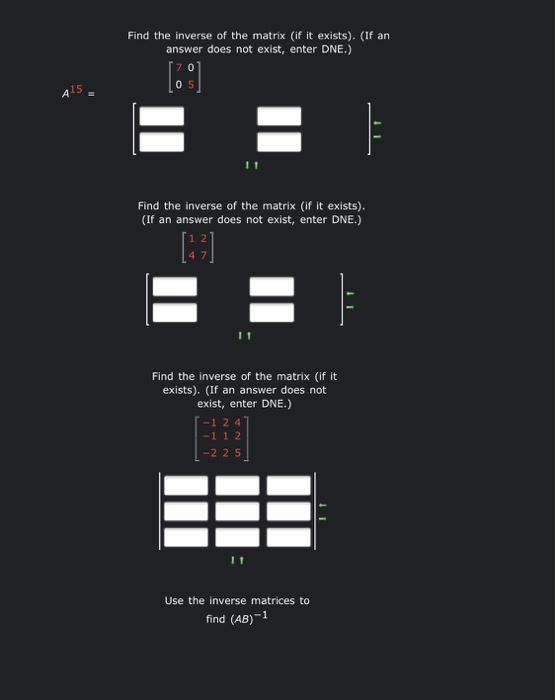3 part question about inverse of matrices. please help!! Find the inverse of the matrix (if it exists). (If an answer does not exist, enter DNE.)  05 415 E Find the inverse of the matrix (if it exists). (If an answer does not exist, enter DNE.) E = Find the inverse of the matrix (if it exists). (If an answer does not exist, enter DNE.) 1-1 2 4 -1 1 2 | -2 25 Use the inverse matrices to...

• ### Examine the product of the two matrices to determine if each is the inverse of the...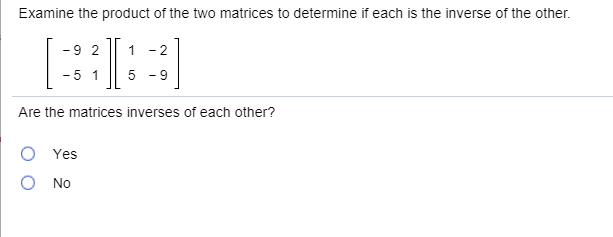Examine the product of the two matrices to determine if each is the inverse of the other. -9211-2 - 5 1 || 5 - Are the matrices inverses of each other? O Ο Yes Νο Identify a 13 and 11, if possible. [ 6 -5 31 | -92 - 6 Select the correct choice below and, if necessary, fill in the answer box(es) to complete your choice O A. 213= and = OB. 213= and any does not exist. O...

• ### Find the​ inverse, if it​ exists, for the given matrix. left bracket Start 2 By 3...

Find the​ inverse, if it​ exists, for the given matrix. left bracket Start 2 By 3 Matrix 1st Row 1st Column 5 2nd Column 3rd Column 5 2nd Row 1st Column 4 2nd Column 3rd Column 5 EndMatrix right bracket 5 5 4 5 Find the inverse. Select the correct choice below​ and, if​ necessary, fill in the answer box to complete your choice. A.The inverse is nothing. ​(Type a​ matrix, using an integer or simplified fraction for each matrix...

• ### 1 -1 -b 1 The inverse of matrix A is (see explanation in Sec. 5.6) and...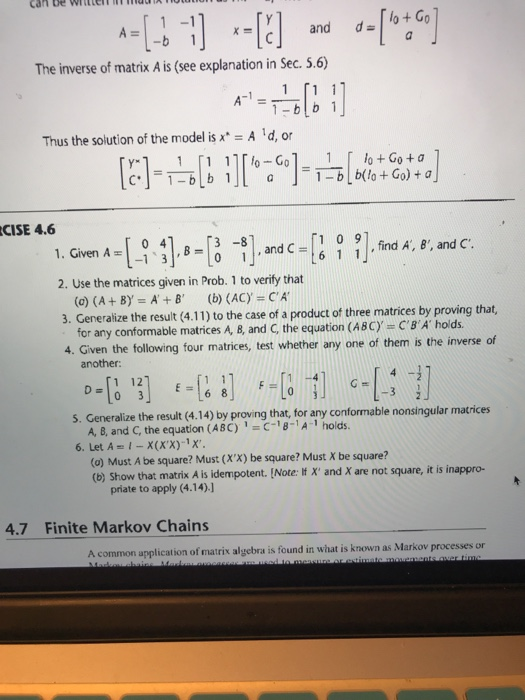1 -1 -b 1 The inverse of matrix A is (see explanation in Sec. 5.6) and d lo+Go A-1 1 1-blb 1 Thus the solution of the model isx A d, or CISE 4.6 1.Given A-B1--B -t].and c-l 1 0 9 ].find A, e-arnd C -1 3 , find A, 8', and C 2. Use the matrices given in Prob. 1 to verify that 3. Generalize the result (4.11) to the case of a product of three matrices by proving...

• ### 14. 0-4 points LarLinAlg8 4.7.042 My Notes O Ask Your Tea Use a software program or a graphing utility to find the transition matrix from B to B', find the transition matrix from B' to B...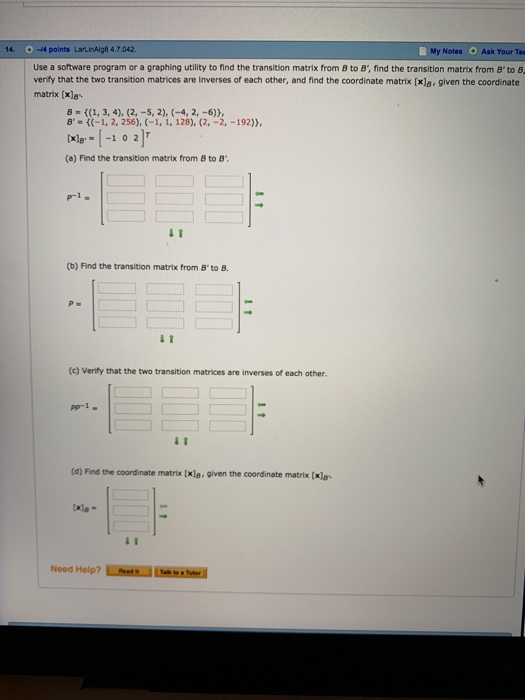14. 0-4 points LarLinAlg8 4.7.042 My Notes O Ask Your Tea Use a software program or a graphing utility to find the transition matrix from B to B', find the transition matrix from B' to B verify that the two transition matrices are Inverses of each other, and find the coordinate matrix [xls, given the coordinate matrix [xle B' = {(-1, 2, 256), (-1, 1. 128), (2,-2,-192)), l102 (a) Find the transition matrix from 8 to B (b) Find the...

• ### 1. On Inverting Matrices, using Gauss-Jordan (a) Consider the following matrix A. If the inverse of...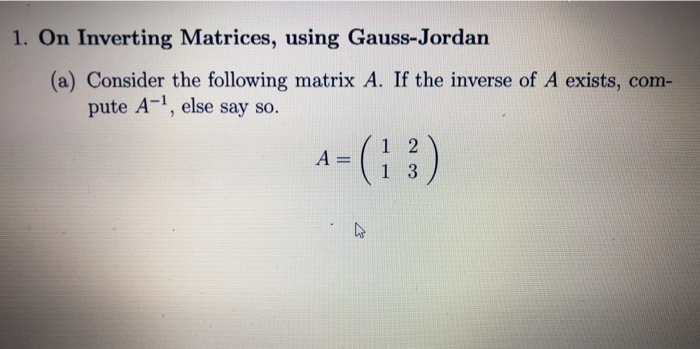1. On Inverting Matrices, using Gauss-Jordan (a) Consider the following matrix A. If the inverse of A exists, com- pute A-1, else say so. A 1 3 INVERSE OF MATRICES 15 (b) Consider the following matrix A. If the inverse of A exists, com- pute A-1, else say so. A-(3) 0 1 (c) Consider the following matrix A. If the inverse of A exists, com- pute A1, else say so. 0 2 (d) Consider the following matrix A. If the...

• ### 6) Find the inverse of each matrix below or explain why no such inverse exists. (10...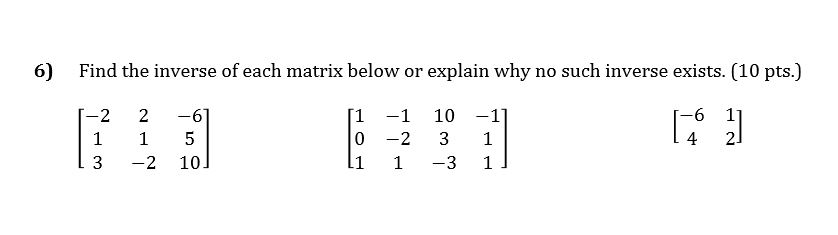6) Find the inverse of each matrix below or explain why no such inverse exists. (10 pts.) 1-2 1 13 2 1 -2 -6] 5 10] [1 0 li -1 -2 1 10 3 -3 -1] 1 1]